In :
import numpy as np
from functools import partial
import matplotlib.pyplot as plt

In :
# To look at the signals with.
class Scope():
def __init__(self, rate):
self.ts = 1 / rate

def __call__(self, samps):
fig, ax = plt.subplots()
ax.grid(True)
xs = np.arange(len(samps)) * self.ts
ax.plot(xs, samps)

In :
# Signal generator.
class Sinusoid():
def __init__(self, rate, freq, amplitude=1.0, phase=0):
self.rate = rate
self.ts = 1 / rate
self.A = amplitude
self.w = 2 * np.pi * freq
self.ph = phase
self.n = 0

def feed(self):
r = self.A * np.sin(self.w * self.n * self.ts + self.ph)
self.n += 1
return r

def __call__(self, samps):
return np.array([self.feed() for i in range(samps)])

In :
def dc(volts, samps):
return np.ones(shape=samps) * volts

In :
class RC_filter():
def __init__(self, sample_freq, RC):
self.k = 1.0 / (RC * sample_freq)
self.y = 0

def feed(self, samp):
self.y += self.k * (samp - self.y)
return self.y

def __call__(self, samps):
return np.array([self.feed(s) for s in samps])

In :
class QuadratureMixer():
def __init__(self, tune, RC, oversamp=100):
self.rate = oversamp * tune
self.tune = tune
self.oversamp = oversamp
self.scope = Scope(self.rate)
self.filt_I = RC_filter(self.rate, RC)
self.filt_Q = RC_filter(self.rate, RC)

def sample_rate(self):
return self.rate

def freq(self):
return self.tune

def cycle(self, sgen):
qtr = self.oversamp // 4
filt_I = self.filt_I
filt_Q = self.filt_Q

I1 = filt_I(sgen(qtr))
Q1 = dc(volts=filt_Q.y, samps=qtr)

I2 = dc(volts=filt_I.y, samps=qtr)
Q2 = filt_Q(sgen(qtr))

I3 = filt_I(-sgen(qtr))
Q3 = dc(volts=filt_Q.y, samps=qtr)

I4 = dc(volts=filt_I.y, samps=qtr)
Q4 = filt_Q(-sgen(qtr))

I = np.concatenate([I1, I2, I3, I4])
Q = np.concatenate([Q1, Q2, Q3, Q4])

return list(zip(I,Q))

def __call__(self, sgen, cycles):
cycles = int(cycles)
vv = np.array([self.cycle(sgen) for i in range(cycles)])
return np.concatenate(vv)
#I = np.concatenate(vv[:, 0])
#Q = np.concatenate(vv[:, 1])
#return I,Q

In :
# Convenient units.
MHz = 1e6; kHz = 1e3; Hz=1
uF = 1e-6; nF = 1e-9; pF = 1e-12
usec = 1e-6; msec = 1e-3; sec = 1
ohms = 1

In :
# Set the radio to tune a 5-MHz signal.
EnsembleRX = partial(QuadratureMixer, RC = 26.5*ohms * 0.047*uF)
rx = EnsembleRX(5.0*MHz)
rate = rx.sample_rate()

In :
# Feed in a carrier 1 kHz above 5 MHz.
# We get two 1-kHz outputs in quadrature, as we should.
IQ = rx(Sinusoid(rate, freq=5*MHz + 1*kHz), cycles=rx.freq()*1*msec)
rx.scope(IQ)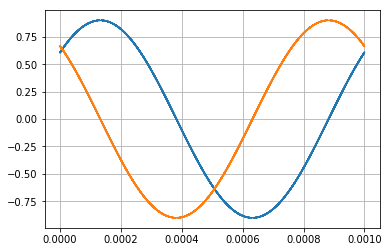In :
# Zoom in on the first few cycles to see the RF ripple on
# the sampling capacitor.
rx.scope(IQ[:500])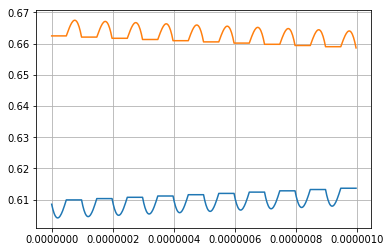In :
# Now feed in a 15-MHz plus 1 kHz signal.
# With the radio tuned to the original 5 MHz we
# get good I and Q, but reversed in phase, and attenuated
# by a factor of 1/3.
IQ = rx(Sinusoid(rate, freq=15*MHz + 1*kHz), cycles=rx.freq()*1*msec)
rx.scope(IQ)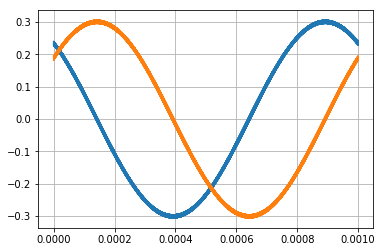In :
# The RF ripple is bigger in absolute terms also, not just
# in relative terms.
rx.scope(IQ[:500])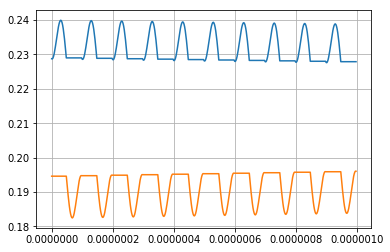The above shows what happens when you use "third-overtone tuning" like is done on the simpler SoftRock Lite with crystal LO.

It's an interesting option to reduce the gain, perhaps preferable to the 14-dB attenuator the Ensemble II uses for the two low bands. In other words, reduce gain by tuning low and get rid of the attenuator.

But I'd have to work through that to be sure this is in fact a gain reduction and not an attenuation.

In [ ]:


In :
# The rest of the plots investigate the rolloff of the signal amplitude as
# you move away from the center frequency.
IQ = rx(Sinusoid(rate, freq=5*MHz + 20*kHz), cycles=rx.freq()*0.1*msec)
rx.scope(IQ)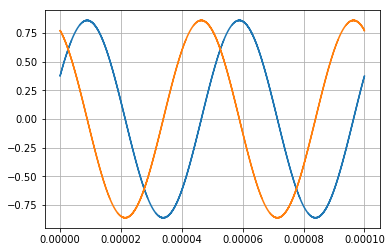In :
IQ = rx(Sinusoid(rate, freq=5*MHz + 40*kHz), cycles=rx.freq()*0.1*msec)
rx.scope(IQ)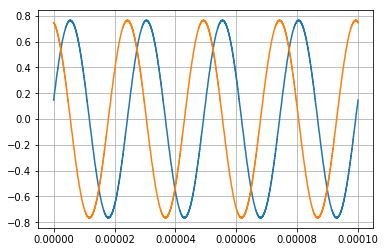In :
IQ = rx(Sinusoid(rate, freq=5*MHz - 40*kHz), cycles=rx.freq()*0.1*msec)
rx.scope(IQ)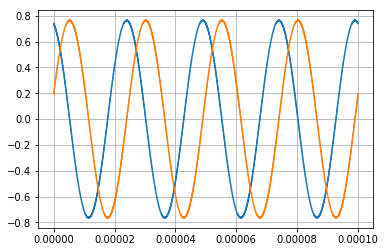In :
# This seems to be about the -3dB point
sgen = Sinusoid(rate, freq=5*MHz + 65*kHz)

In :
IQ = rx(sgen, cycles=rx.freq()*0.1*msec)
rx.scope(IQ)
print(IQ.max())

0.635110667233397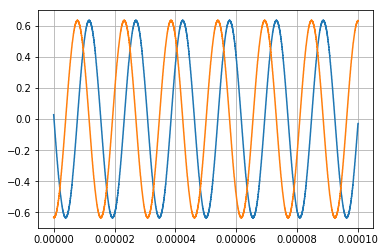In :
IQ = rx(Sinusoid(rate, freq=5*MHz + 100*kHz), cycles=rx.freq()*0.01*msec)
rx.scope(IQ)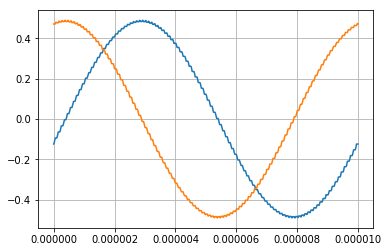Notes:

• Here is the schematic.
• For the I component to be truly in phase the received signal needs to be phase delayed by 45 degrees, as done in the signal generator. This is okay, because there is nothing magic about the phase of the local oscillator--it just starts whenever you turn it on.
• You can see that with this 45-degree phase delay, the I half of the detector is centered on the two peaks, and the Q side of the detector is centered on the two zeros.
• The sound card sees many copies of these single-cycle waveforms during its audio sample. The average that it sees is indicated in one of the plots.
• The small amount of ripple you see at the detector output passes through the op amp gain stage before being passed on to the sound card, and so the bandwidth there would likely attenuate the ripple some.
• The amount of ripple is really determined by the source impedance and the sampling capacitor, which is really just a filter capacitor. You can make it larger and get less ripple, but then you'd increase the time to achieve steady state. I believe that time is on the order of several time constants for the filter. With the parts shown, the time constant is about 12.5 microseconds.
• I think this also sets the bandwidth that you have available for sampling with the sound card. If you have a fancy high-bandwidth sound card these component values might be limiting. The data above indicates that the -3dB point is about 66 kHz off of center. But that is in both directions, so the bandwidth would seem to be about 132 kHz.
In [ ]: